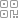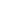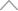6 2 9 1 1 1 8

# 用Python可视化分析全球火山分布，发现了这些有趣的现象## 准备工作

import pandas as pd import folium.plugins as plugins import folium df_volcano = pd.read_csv("volcano.csv") df_volcano.head()

outputdf_volcano.columns

output

Index(['volcano_number', 'volcano_name', 'primary_volcano_type', 'last_eruption_year', 'country', 'region', 'subregion', 'latitude', 'longitude', 'elevation', 'tectonic_settings', 'evidence_category', 'major_rock_1', 'major_rock_2', 'major_rock_3', 'major_rock_4', 'major_rock_5', 'minor_rock_1', 'minor_rock_2', 'minor_rock_3', 'minor_rock_4', 'minor_rock_5', 'population_within_5_km', 'population_within_10_km', 'population_within_30_km', 'population_within_100_km'], dtype='object')

## 全球火山带的分布可视化

volcano_map = folium.Map() # 将每一行火山的数据添加进来 for i in range(0, df_volcano.shape): volcano = df_volcano.iloc[i] folium.Marker([volcano['latitude'], volcano['longitude']], popup=volcano['volcano_name']).add_to(volcano_map) volcano_map

outputfig, (ax1, ax2) = plt.subplots(1, 2, figsize=(16, 4)) volcano_country = pd.DataFrame(df_volcano.groupby(['country']).size()).sort_values(0, ascending=True) volcano_country.columns = ['Count'] volcano_country.tail(10).plot(kind='barh', legend=False, ax=ax1) ax1.set_title('Number of Volcanoes per Country') ax1.set_ylabel('Country') ax1.set_xlabel('Count') volcano_region = pd.DataFrame(df_volcano.groupby(['region']).size()).sort_values(0, ascending=True) volcano_region.columns = ['Count'] volcano_region.tail(10).plot(kind='barh', legend=False, ax=ax2) ax2.set_title('Number of Volcanoes per Region') ax2.set_ylabel('Region') ax2.set_xlabel('Count') plt.tight_layout() plt.show()

output## 全球火山带的分布可视化优化

volcano_map = folium.Map(zoom_start=10) groups = folium.FeatureGroup('') # 将每一行火山的数据添加进来 for i in range(0, df_volcano.shape): volcano = df_volcano.iloc[i] groups.add_child(folium.CircleMarker([volcano['latitude'], volcano['longitude']], popup=volcano['volcano_name'], radius=3, color='blue', fill=True, fill_color='blue',fill_opacity=0.8)) volcano_map.add_child(groups) volcano_map.add_child(folium.LatLngPopup())

output## 地图可视化实战

import folium.plugins as plugins import folium m = folium.Map([-21.178986, -175.198242], zoom_start=10, control_scale=True, width='80%') m

output## 在地图上打上标记

m = folium.Map([-21.178986, -175.198242], zoom_start=12, control_scale=True, width='80%') folium.Circle(location = [-21.177986, -175.199242], radius = 1500, color = "purple").add_to(m) m

outputm = folium.Map([-21.178986, -175.198242], zoom_start=12, control_scale=True, width='80%') folium.Circle(location = [-21.177986, -175.199242], radius = 1500, color = "purple", fill = True, fill_color = "red").add_to(m) m

output## 深远影响## 新知精选

IT研发▪2021年度十佳创作者

### 优选课程 新知学院

•扫码下载商业新知APP~

•回到顶部